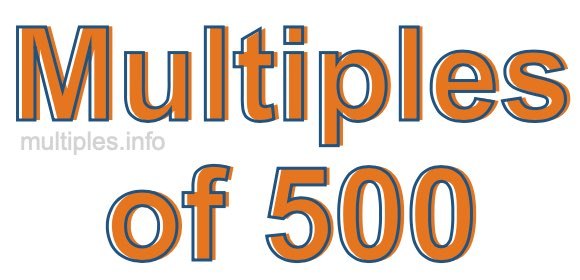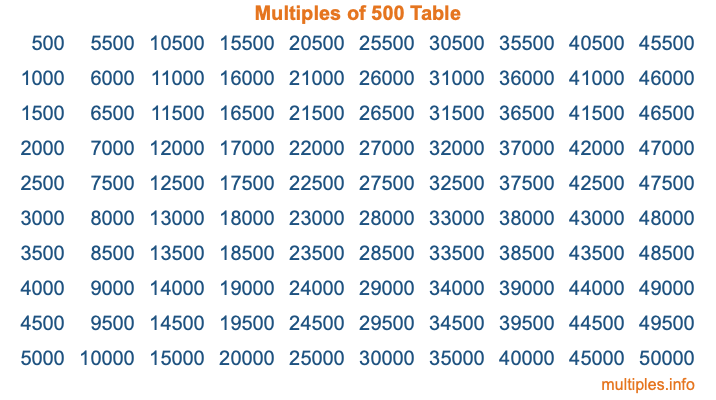Multiples of 500Welcome to the Multiples of 500 page. Here we will first teach you everything you will ever need to know about the multiples of 500, and then give you a study guide summary of everything we taught you to make sure you remember it all. Use this page to look up facts and learn information about the multiples of 500. This page will make you a multiples of five hundred expert!

Definition of Multiples of 500
Multiples of 500 are all the numbers that when divided by 500 equal an integer. Each of the multiples of 500 are called a multiple. A multiple of 500 is created by multiplying 500 by an integer.

Therefore, to create a list of multiples of 500, you start with 1 multiplied by 500, then 2 multiplied by 500, then 3 multiplied by 500, and so on for as long as you want. Thus, the list of the first five multiples of 500 is 500, 1000, 1500, 2000, and 2500. To see a larger list of multiples of 500, see the printable image of Multiples of 500 further down on this page. We also have a category where you can choose any nth multiple of 500.

Multiples of 500 Checker
The Multiples of 500 Checker below checks to see if any number of your choice is a multiple of 500. In other words, it checks to see if there is any number (integer) that when multiplied by 500 will equal your number. To do that, we divide your number by 500. If the the quotient is an integer, then your number is a multiple of 500.

Is  a multiple of 500?

Least Common Multiple of 500 and ...
A Least Common Multiple (LCM) is the lowest multiple that two or more numbers have in common. This is also called the smallest common multiple or lowest common multiple and is useful to know when you are adding our subtracting fractions. Enter one or more numbers below (500 is already entered) to find the LCM.

Check out our LCM Calculator if you need more details about the Least Common Multiple or if you need the LCM for different numbers for adding and subtraction fractions.

nth Multiple of 500
As we stated above, 500 is the first multiple of 500, 1000 is the second multiple of 500, 1500 is the third multiple of 500, and so on. Enter a number below to find the nth multiple of 500.

th multiple of 500

Multiples of 500 vs Factors of 500
500 is a multiple of 500 and a factor of 500, but that is where the similarities end. All postive multiples of 500 are 500 or greater than 500. All positive factors of 500 are 500 or less than 500.

Below is the beginning list of multiples of 500 and the factors of 500 so you can compare:

Multiples of 500: 500, 1000, 1500, 2000, 2500, etc.

Factors of 500: 1, 2, 4, 5, 10, 20, 25, 50, 100, 125, 250, 500

As you can see, the multiples of 500 are all the numbers that you can divide by 500 to get a whole number. The factors of 500, on the other hand, are all the whole numbers that you can multiply by another whole number to get 500.

It's also interesting to note that if a number (x) is a factor of 500, then 500 will also be a multiple of that number (x).

Multiples of 500 vs Divisors of 500
The divisors of 500 are all the integers that 500 can be divided by evenly. Below is a list of the divisors of 500.

Divisors of 500: 1, 2, 4, 5, 10, 20, 25, 50, 100, 125, 250, 500

The interesting thing to note here is that if you take any multiple of 500 and divide it by a divisor of 500, you will see that the quotient is an integer.

Multiples of 500 Table
Below is an image of the first 100 multiples of 500 in a table. The table is in chronological order, column by column. The first column has the first ten multiples of 500, the second column has the next ten multiples of 500, and so on.The Multiples of 500 Table is also referred to as the 500 Times Table or Times Table of 500. You are welcome to print out our table for your studies.

Negative Multiples of 500
Although not often discussed or needed in math, it is worth mentioning that you can make a list of negative multiples of 500 by multiplying 500 by -1, then by -2, then by -3, and so on, to get the following list of negative multiples of 500:

-500, -1000, -1500, -2000, -2500, etc.

Multiples of 500 Summary
Below is a summary of important Multiples of 500 facts that we have discussed on this page. To retain the knowledge on this page, we recommend that you read through the summary and explain to yourself or a study partner why they hold true.

There are an infinite number of multiples of 500.

A multiple of 500 divided by 500 will equal a whole number.

500 divided by a factor of 500 equals a divisor of 500.

The nth multiple of 500 is n times 500.

The largest factor of 500 is equal to the first positive multiple of 500.

500 is a multiple of every factor of 500.

500 is a multiple of 500.

A multiple of 500 divided by a divisor of 500 equals an integer.

500 divided by a divisor of 500 equals a factor of 500.

Any integer times 500 will equal a multiple of 500.

Multiples of a Number
Here you can get the multiples of another number, all with the same attention to detail as we did for multiples of 500 on this page.

Multiples of
Multiples of 501
Did you find our page about multiples of five hundred educational? Do you want more knowledge? Check out the multiples of the next number on our list!